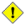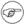# 8.1. Operators

assignment

variable assignment

Initializing or changing the value of a variable

=

All-purpose assignment operator, which works for both arithmetic and string assignments.

 ``` 1 var=27 2 category=minerals # No spaces allowed after the "=".```Do not confuse the "=" assignment operator with the = test operator.

 ``` 1 # = as a test operator 2  3 if [ "\$string1" = "\$string2" ] 4 then 5  command 6 fi 7  8 # if [ "X\$string1" = "X\$string2" ] is safer, 9 #+ to prevent an error message should one of the variables be empty. 10 # (The prepended "X" characters cancel out.)```

arithmetic operators

+

plus

-

minus

*

multiplication

/

division

**

exponentiation

 ``` 1 # Bash, version 2.02, introduced the "**" exponentiation operator. 2  3 let "z=5**3" 4 echo "z = \$z" # z = 125```

%

modulo, or mod (returns the remainder of an integer division operation)

 ``` bash\$ expr 5 % 3 2 ```
5/3 = 1 with remainder 2

This operator finds use in, among other things, generating numbers within a specific range (see Example 9-26 and Example 9-29) and formatting program output (see Example 26-15 and Example A-6). It can even be used to generate prime numbers, (see Example A-16). Modulo turns up surprisingly often in various numerical recipes.

Example 8-1. Greatest common divisor

 ``` 1 #!/bin/bash 2 # gcd.sh: greatest common divisor 3 # Uses Euclid's algorithm 4  5 # The "greatest common divisor" (gcd) of two integers 6 #+ is the largest integer that will divide both, leaving no remainder. 7  8 # Euclid's algorithm uses successive division. 9 # In each pass, 10 #+ dividend <--- divisor 11 #+ divisor <--- remainder 12 #+ until remainder = 0. 13 #+ The gcd = dividend, on the final pass. 14 # 15 # For an excellent discussion of Euclid's algorithm, see 16 #+ Jim Loy's site, http://www.jimloy.com/number/euclids.htm. 17  18  19 # ------------------------------------------------------ 20 # Argument check 21 ARGS=2 22 E_BADARGS=65 23  24 if [ \$# -ne "\$ARGS" ] 25 then 26  echo "Usage: `basename \$0` first-number second-number" 27  exit \$E_BADARGS 28 fi 29 # ------------------------------------------------------ 30  31  32 gcd () 33 { 34  35  dividend=\$1 # Arbitrary assignment. 36  divisor=\$2 #! It doesn't matter which of the two is larger. 37  # Why not? 38  39  remainder=1 # If uninitialized variable used in loop, 40  #+ it results in an error message 41  #+ on the first pass through loop. 42  43  until [ "\$remainder" -eq 0 ] 44  do 45  let "remainder = \$dividend % \$divisor" 46  dividend=\$divisor # Now repeat with 2 smallest numbers. 47  divisor=\$remainder 48  done # Euclid's algorithm 49  50 } # Last \$dividend is the gcd. 51  52  53 gcd \$1 \$2 54  55 echo; echo "GCD of \$1 and \$2 = \$dividend"; echo 56  57  58 # Exercise : 59 # -------- 60 # Check command-line arguments to make sure they are integers, 61 #+ and exit the script with an appropriate error message if not. 62  63 exit 0```

+=

"plus-equal" (increment variable by a constant)

let "var += 5" results in var being incremented by 5.

-=

"minus-equal" (decrement variable by a constant)

*=

"times-equal" (multiply variable by a constant)

let "var *= 4" results in var being multiplied by 4.

/=

"slash-equal" (divide variable by a constant)

%=

"mod-equal" (remainder of dividing variable by a constant)

Arithmetic operators often occur in an expr or let expression.

Example 8-2. Using Arithmetic Operations

 ``` 1 #!/bin/bash 2 # Counting to 11 in 10 different ways. 3  4 n=1; echo -n "\$n " 5  6 let "n = \$n + 1" # let "n = n + 1" also works. 7 echo -n "\$n " 8  9  10 : \$((n = \$n + 1)) 11 # ":" necessary because otherwise Bash attempts 12 #+ to interpret "\$((n = \$n + 1))" as a command. 13 echo -n "\$n " 14  15 (( n = n + 1 )) 16 # A simpler alternative to the method above. 17 # Thanks, David Lombard, for pointing this out. 18 echo -n "\$n " 19  20 n=\$((\$n + 1)) 21 echo -n "\$n " 22  23 : \$[ n = \$n + 1 ] 24 # ":" necessary because otherwise Bash attempts 25 #+ to interpret "\$[ n = \$n + 1 ]" as a command. 26 # Works even if "n" was initialized as a string. 27 echo -n "\$n " 28  29 n=\$[ \$n + 1 ] 30 # Works even if "n" was initialized as a string. 31 #* Avoid this type of construct, since it is obsolete and nonportable. 32 # Thanks, Stephane Chazelas. 33 echo -n "\$n " 34  35 # Now for C-style increment operators. 36 # Thanks, Frank Wang, for pointing this out. 37  38 let "n++" # let "++n" also works. 39 echo -n "\$n " 40  41 (( n++ )) # (( ++n ) also works. 42 echo -n "\$n " 43  44 : \$(( n++ )) # : \$(( ++n )) also works. 45 echo -n "\$n " 46  47 : \$[ n++ ] # : \$[ ++n ]] also works 48 echo -n "\$n " 49  50 echo 51  52 exit 0```Integer variables in Bash are actually signed long (32-bit) integers, in the range of -2147483648 to 2147483647. An operation that takes a variable outside these limits will give an erroneous result.

 ``` 1 a=2147483646 2 echo "a = \$a" # a = 2147483646 3 let "a+=1" # Increment "a". 4 echo "a = \$a" # a = 2147483647 5 let "a+=1" # increment "a" again, past the limit. 6 echo "a = \$a" # a = -2147483648 7  # ERROR (out of range)```

As of version 2.05b, Bash supports 64-bit integers.Bash does not understand floating point arithmetic. It treats numbers containing a decimal point as strings.

 ``` 1 a=1.5 2  3 let "b = \$a + 1.3" # Error. 4 # t2.sh: let: b = 1.5 + 1.3: syntax error in expression 5 # (error token is ".5 + 1.3") 6  7 echo "b = \$b" # b=1```

Use bc in scripts that that need floating point calculations or math library functions.

bitwise operators. The bitwise operators seldom make an appearance in shell scripts. Their chief use seems to be manipulating and testing values read from ports or sockets. "Bit flipping" is more relevant to compiled languages, such as C and C++, which provide direct access to system hardware.

bitwise operators

<<

bitwise left shift (multiplies by 2 for each shift position)

<<=

"left-shift-equal"

let "var <<= 2" results in var left-shifted 2 bits (multiplied by 4)

>>

bitwise right shift (divides by 2 for each shift position)

>>=

"right-shift-equal" (inverse of <<=)

&

bitwise and

&=

"bitwise and-equal"

|

bitwise OR

|=

"bitwise OR-equal"

~

bitwise negate

!

bitwise NOT

^

bitwise XOR

^=

"bitwise XOR-equal"

logical operators

&&

and (logical)

 ``` 1 if [ \$condition1 ] && [ \$condition2 ] 2 # Same as: if [ \$condition1 -a \$condition2 ] 3 # Returns true if both condition1 and condition2 hold true... 4  5 if [[ \$condition1 && \$condition2 ]] # Also works. 6 # Note that && operator not permitted within [ ... ] construct.```&& may also, depending on context, be used in an and list to concatenate commands.
||

or (logical)

 ``` 1 if [ \$condition1 ] || [ \$condition2 ] 2 # Same as: if [ \$condition1 -o \$condition2 ] 3 # Returns true if either condition1 or condition2 holds true... 4  5 if [[ \$condition1 || \$condition2 ]] # Also works. 6 # Note that || operator not permitted within [ ... ] construct.```Bash tests the exit status of each statement linked with a logical operator.

Example 8-3. Compound Condition Tests Using && and ||

 ``` 1 #!/bin/bash 2  3 a=24 4 b=47 5  6 if [ "\$a" -eq 24 ] && [ "\$b" -eq 47 ] 7 then 8  echo "Test #1 succeeds." 9 else 10  echo "Test #1 fails." 11 fi 12  13 # ERROR: if [ "\$a" -eq 24 && "\$b" -eq 47 ] 14 #+ attempts to execute ' [ "\$a" -eq 24 ' 15 #+ and fails to finding matching ']'. 16 # 17 # Note: if [[ \$a -eq 24 && \$b -eq 24 ]] works. 18 # The double-bracket if-test is more flexible 19 #+ than the single-bracket version. 20 # (The "&&" has a different meaning in line 17 than in line 6.) 21 # Thanks, Stephane Chazelas, for pointing this out. 22  23  24 if [ "\$a" -eq 98 ] || [ "\$b" -eq 47 ] 25 then 26  echo "Test #2 succeeds." 27 else 28  echo "Test #2 fails." 29 fi 30  31  32 # The -a and -o options provide 33 #+ an alternative compound condition test. 34 # Thanks to Patrick Callahan for pointing this out. 35  36  37 if [ "\$a" -eq 24 -a "\$b" -eq 47 ] 38 then 39  echo "Test #3 succeeds." 40 else 41  echo "Test #3 fails." 42 fi 43  44  45 if [ "\$a" -eq 98 -o "\$b" -eq 47 ] 46 then 47  echo "Test #4 succeeds." 48 else 49  echo "Test #4 fails." 50 fi 51  52  53 a=rhino 54 b=crocodile 55 if [ "\$a" = rhino ] && [ "\$b" = crocodile ] 56 then 57  echo "Test #5 succeeds." 58 else 59  echo "Test #5 fails." 60 fi 61  62 exit 0```

The && and || operators also find use in an arithmetic context.

 ``` bash\$ echo \$(( 1 && 2 )) \$((3 && 0)) \$((4 || 0)) \$((0 || 0)) 1 0 1 0 ```

miscellaneous operators

,

comma operator

The comma operator chains together two or more arithmetic operations. All the operations are evaluated (with possible side effects), but only the last operation is returned.

 ``` 1 let "t1 = ((5 + 3, 7 - 1, 15 - 4))" 2 echo "t1 = \$t1" # t1 = 11 3  4 let "t2 = ((a = 9, 15 / 3))" # Set "a" and calculate "t2". 5 echo "t2 = \$t2 a = \$a" # t2 = 5 a = 9```

The comma operator finds use mainly in for loops. See Example 10-12.